• 求两个数最小公倍数最大公约数C++,编程环境在VS2010下以实验。
• 最大公因数最小公倍数的数学公式算法如下： 最大公因数：辗转相除法（详情请百度） 就是形如两个整数n,m并且n>m; 则可以通过n/m得到一个余数是否为零判断m是否为n和m的最大公因数， 如果不相等，则将m作为被除...

一.最大公因数和最小公倍数的数学公式算法如下：

最大公因数：辗转相除法（详情请百度） 就是形如两个整数n,m并且n>m; 则可以通过n/m得到一个余数是否为零判断m是否为n和m的最大公因数， 如果不相等，则将m作为被除数，余数作为除数在进行上述操作，直到余数等于零，则那个除数就为两个整数的最大公因数。
这里有重复的操作，不断的相除： 除数变为下一个的被除数，余数变为下一个的除数（迭代的表达关系式）
迭代停止条件：这一个迭代函数里的除数也就是上一个里的余数为0；
通过以上就可以写出一个迭代函数；
最小公倍数： 还是n和m，用求得的最大公因数x,则最小公倍数为 n*m/x;
二.代码如下：
#include <iostream>
using namespace std;
int gcd(int n, int m);
int lcm(int n, int m);
int main() {
int n, m;
cin>>n>>m;
cout<<gcd(n,m)<<endl;
cout<<lcm(n,m)<<endl;

return 0;
}
int gcd(int n, int m) {     //目的是让n作为被除数，m作为除数，得到n/m和一个余数
if (m <= n) {
// 请在这里继续完成 gcd 函数
return m==0?n:gcd(m,n%m);  //如果m不等于0，则上一个n/m得到的余数不为0
//然后将除数作为下一个的被除数，将余数作为下一个的除数
} else {               //n<m的话肯定不能让n作为被除数所以要调换一下
return gcd(m, n);
}

}
int lcm(int n, int m) {
// 请在这里继续完成 lcm 函数
return n*m/gcd(n,m);
}

展开全文算法
• C++计算两个数最大公因数最小公倍数
//Author:PanDaoxi
#include <iostream>
using namespace std;
int mcf(int x,int y){ //最大公因数
int maxn,a,k=0,big=(x>y?x:y);
for(int i=1;i<=big;i++){
if(x%i==0&&y%i==0) a[k++]=i;
}
maxn=a;
for(int i=0;i<k;i++){
if(maxn<a[i]) maxn=a[i];
}
return maxn;
}
bool coprime(int x,int y){ // 是否互质
int n=mcf(x,y);
if(n==1) return true;
else return false;
}
int lcm(int x,int y,int z){ // 最小公倍数
int sum=1,n=0,minn,b,k=0,big=(x>y?x:y);
if(coprime(x,y)) return x*y;
int c=x,d=y;
n=mcf(c,d);
while(coprime(c,d)==false&&n!=1){
n=mcf(c,d);
c/=n;
d/=n;
b[k++]=n;
}
sum=c*d;
for(int i=0;i<k;i++){
sum*=b[i];
}
return sum;
}

int main(){
int a,b;
cin>>a>>b;
int x=mcf(a,b);
int y=lcm(a,b,x);
cout<<"最大公因数："<<x<<endl<<"最小公倍数："<<y<<endl;
return 0;
}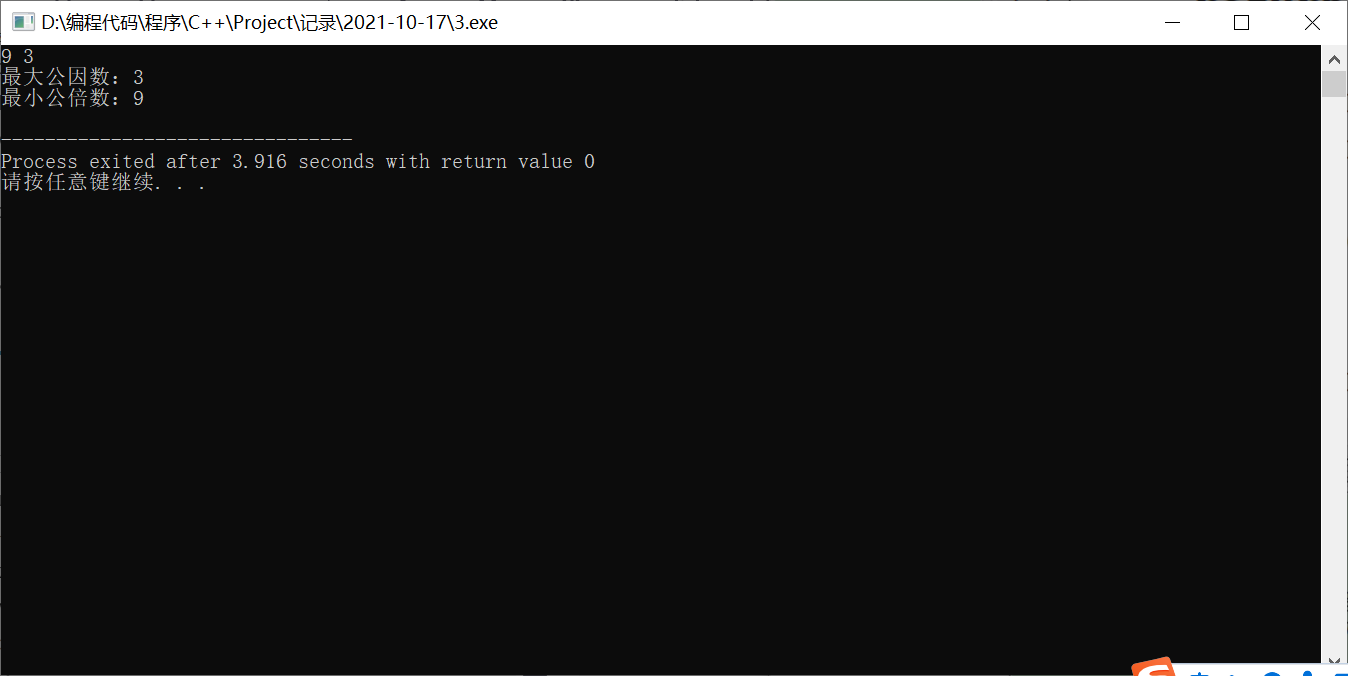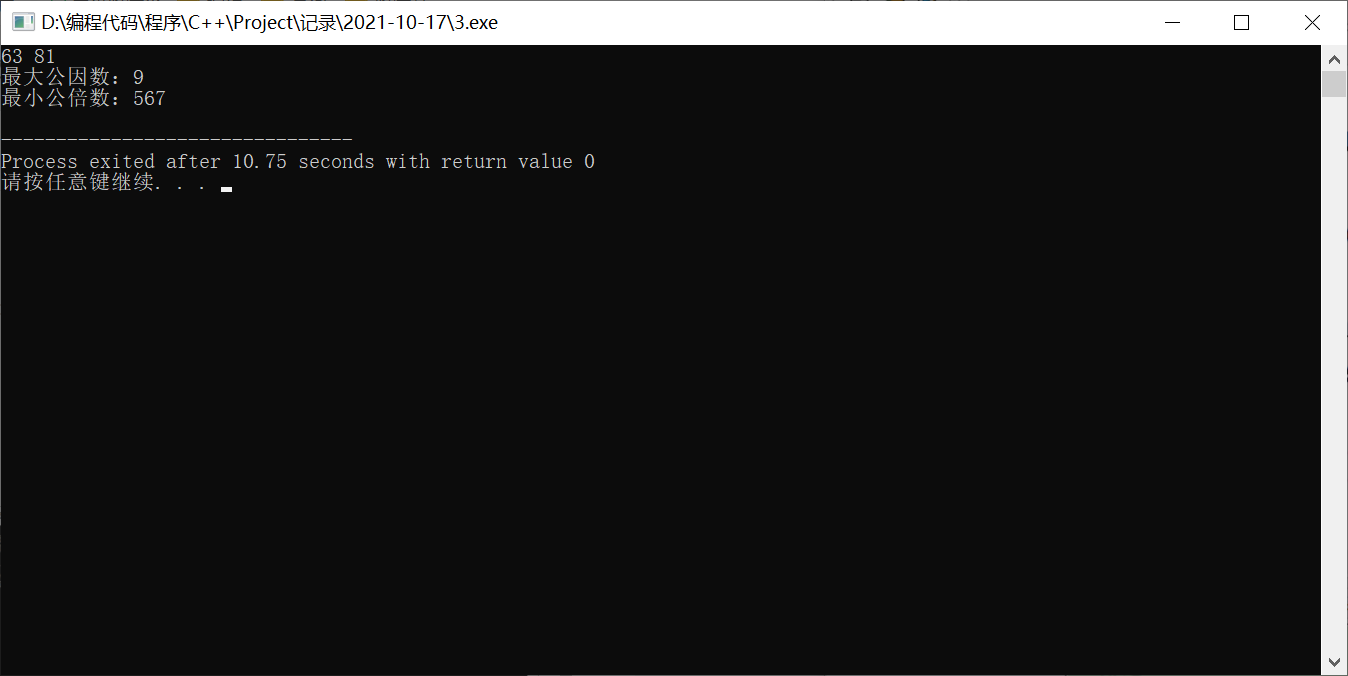展开全文• n个最大公约数和最小公倍数。 二、解题思路 1、首先我们可以利用辗转相除法编写一个函数gcd(int a,int b)来两个数最大公约数，然后将输入的n个放进数组a中，编写一个函数GCD（int a[],int n）...
一、问题描述  求n个数的最大公约数和最小公倍数。 二、解题思路 1、首先我们可以利用辗转相除法编写一个函数gcd(int a,int b)来求出两个数的最大公约数，然后将输入的n个数放进数组a中，编写一个函数GCD（int a[],int n）函数利用for循环来依次求解每两个数的最大公约数，最后得到n个数的最大公约数。 2、求出两个数的最大公约数后，若k是a和b的最大公约数，那么a和b的最小公倍数为ab/k。编写函数lcm（int a,int b）求出两个数的最小公倍数，利用循环结构遍历一次数组即可求出n个数的最小公倍数。 3、在进行数据的正确性验证时，我们可以编写一个检验函数inspect(int a[],int n)来判断输入的数字中是否存在0，若存在则重新输入。 三、主要代码 1、检验函数int inspect(int a[],int n) //判断输入的数字中是否含有0  { for(int i=0;i<n;i++)  { if(a[i]==0)  {cout<<“输入的数字中含有0，错误！”;  return 1;  }  }  return 0 ; } 2、求n个数的最大公约数 Int gcd(int a,int b) //求两个数的最大公约数 { int temp; if(a<b) //使得a必须大于b { temp=a;  a=b;  b=temp; }  while(b!=0) //通过循环求两个数的余数，直到余数为0  { temp=a%b; a=b; b=temp; }  return(a); } Int GCD(int a[],int n) //求n个数的最大公约数 { int i; int c=a;  for (i=1;i<n;i++) //利用for循环 调用求两个最大公约数的函数来求n个数得最大公约数 { c=gcd(c,a[i]);}  return c;} 3、求n个数的最小公倍数 Int lcm(int x,int y) //求两个数的最小公倍数 { return xy/gcd(x,y); } Int LCM(int a[],int n) //求n个数的最小公倍数 { int i; int s=1;  for (i=0;i<n;i++) {s=lcm(s,a[i]);}  return s;} 四、算法设计思路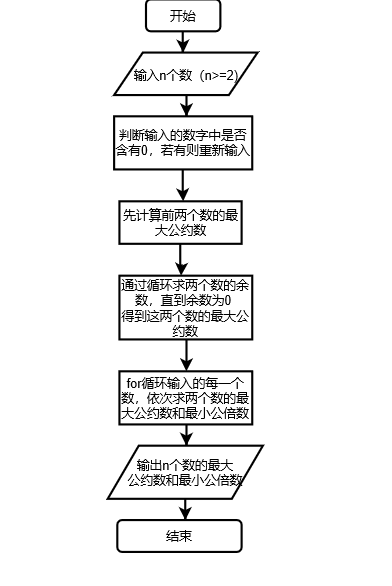五、调试，测试及运行结果 输入数字，结果输出个数的最大公约数和最小公倍数。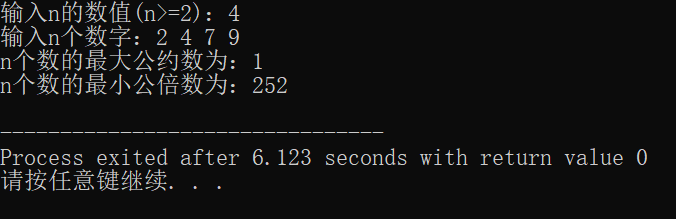六、经验总结 刚开始看见这个题目的时候，我只想到用辗转相除法来求两个数的最大公约数和最小公倍数。然后网上查询以后知道要再利用一个for循环来把输入的数字保存到数组里遍历，先求前两个数的最大公约数，再用前两个数的最大公约数来和第三个数求它们的最大公约数，就这样依次进行，最后求出n个数的最大公约数和最小公倍数。从这个题目中我也学会了如何将数学思维转换成编程语言。 代码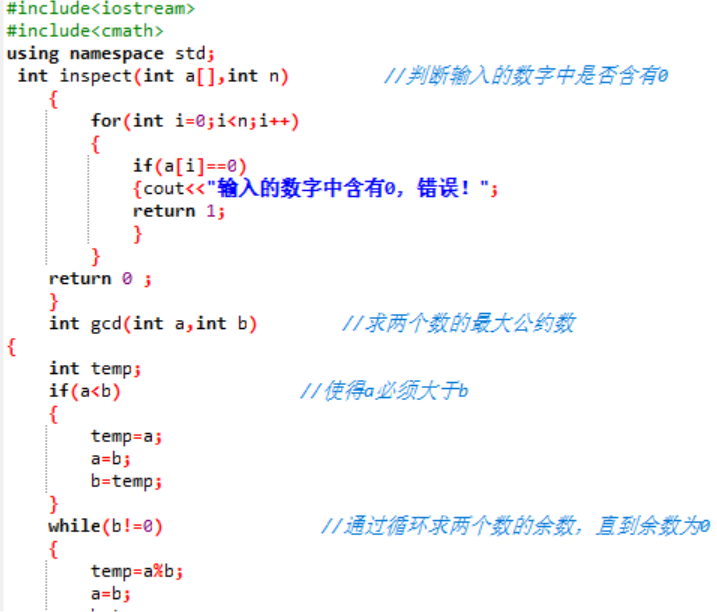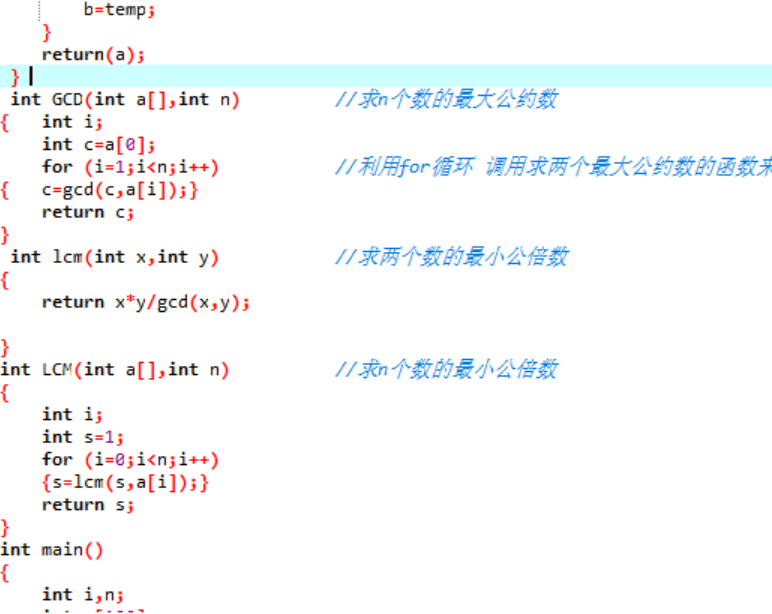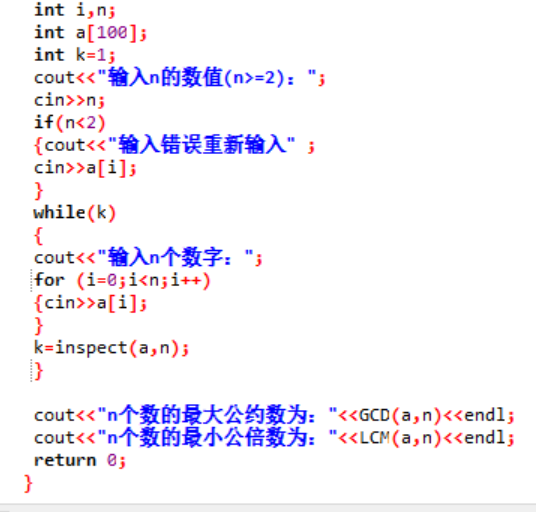展开全文• 求两个数最小公倍数最大公约数C++源码和程序
• 对于每组数据n1和n2,计算最小公倍数，每计算结果应单独占一行。 输入样例： 6 5 18 12 输出样例： 30 36 #include<iostream> using namespace std; //声明gcd函数，该函数用来计算两数最大公约数 int...
输入描述： 输入数据含有不多于50对的数据，每对数据由两个整数（0<n1,n2<100 000)组成 输出描述： 对于每组数据n1和n2,计算最小公倍数，每个计算结果应单独占一行。 输入样例： 6 5 18 12 输出样例： 30 36
#include<iostream>
using namespace std;
//声明gcd函数，该函数用来计算两数的最大公约数
int gcd(int ,int);
int main(int argc ,char *argv[]){
int x,y;
while(cin>>x>>y){
//最小公倍数=X*Y/gcd（x,y)
//因为两数先乘会产生很大的数，可能会超过整数的表示范围
//所以用：最小公倍数=X/gcd(x,y)*Y
cout<<x/gcd(x,y)*y <<endl;
}
return 0;
}
int gcd(int x,int y){
while(x!=y){
if(x>y) x=x-y;
else
y=y-x;
}
return x;
}

展开全文ACM
• 本人最近学习了三种求解两个数最大公因数的方法，第一种是辗转相除法，第二种是相减法，第三种是穷举法 方法一：辗转相除法： 首先判断m是否小于n，不然交换两个值，始终保持m永远是最大的值，余d=m%n,判断余数...
• c++ 计算两个数最大公因数最小公倍数 源代码如下： #include "stdafx.h" #include<iostream> using namespace std; int calc(int m, int n) { int r,a=0; if (n > m) { r = m; m = n; n = r...
• 分享一个大牛的人工智能教程。... * 求两个自然数的最小公倍数 - C++ - by Chimomo * * Answer：最小公倍数 = 两的乘积 / 最大公约数 */ #include &lt;iostream&gt; #include &lt;casser...算法
• 编写函数，递归求两个整数的最大公因数。编写主函数，输入两个整数，调用函数求最大公因数，在主函数中输出。 输入： 两个正整数。 输出： 一个整数。 输入样例：24 42 输出样例： 6 下面代码 #include <...算法 递归法
• 函数版 #include using namespace std; int gys(int a,int b) { while(b) { int r=a%b; a=b; b=r; } return a; } int gbs(int a,int b) { return a/gys(a,b)*b;...请随手点赞哦n( ≧▽≦ )nc 函数 编程
• 输入两个数m,n,求最小公倍数 首先 m , n 比大小，假设得出 m 较大，然后令 i 从 m 开始递增的整数除以 n和m ，直到输出余数为零 #include<stdio.h> int main(){ int m,n,t,i; scanf("%d %d",&m,&n);...c语言
• c++实现数最大公倍数 1、什么是最大公倍数？ 最大公因数就是一个两个数的共同因数,并且是最大的。比如说45 和 15 这两个数，其最大公倍数就是15本身。 2、递归
• 最小公倍数等于要求的两个数的乘积除以它们的最大公约数 比如说，x和y的最小公倍数： lcm(x,y) = x * y / gcd(x,y) 最小公倍数 Least Common Multiple（LCM） 最大公约数 Greatest Common Divisor(GCD) 代码如下：...算法 函数式编程
• 看下面的一段代码 int(int x,int y) { int temp;  while(x)  { temp=x; x=y%x; y=temp;}  }  return y;  }  这是x,y最大公约数的代码，那么我们应该怎么理解他的算法精髓呢。
• 求两个正整数的最大公约数和最小公倍数。 基本要求：1.程序风格良好(使用自定义注释模板)，两种以上算法解决最大公约数问题，提供友好的输入输出。 提高要求：1.三种以上算法解决两个正整数最大公约数问题。 2....java
• 求两个数最大公约数和最小公倍数 方法：最大公约数使用辗转相除法最小公倍数等于两个数的乘积/最大公约数c语言
• 输入两个正整数m和n，最大公约数和最小公倍数 #include "stdafx.h" #include using namespace std; int _tmain(int argc, _TCHAR* argv[]) { int m,n,i,j,gys,gbs; cin>>m; cin>>n; //求最大...
• C++求最小公倍数最大公因数 最近自学了vector感觉比纯穷举法求最小公倍数效率高。用得初中算最小公倍数的算法，顺便练练vector容器 #include<string> #include<iostream> #include<vector> #...
• 第一行输入两个数据n和m并且在第二行输出这两个数字的最大公因数。 二、代码 1.代码的实现 代码如下（示例）： //辗转相除最大公因数 #include<bits/stdc++.h> using namespace std; int gcd(int a,int b)...
• 这里采用欧几里得算法： 递归： #include<iostream> using namespace std; int get(int a,int b) { if(a==0) return b; else get(b%a,a); } int main() { int a,b; while(cin>>...算法
• #include <iostream> using namespace std; int main(){ int m,n,t,flag; cout<<"请输入两个数："<<endl;..."注意，最大公约数和最小公倍数都是对非0而言的,请重新输入！"; }
• 输入两个正整数m和n，最大公约数和最小公倍数 #include "stdafx.h" #include&lt;iostream&gt; using namespace std; int _tmain(int argc, _TCHAR* argv[]) { int m,n,t; cout&lt;&...
• 有A和B两个整数最大公因数： 在A和B的因数中能同时整除A和B的最大。 法：辗转相除法（欧几里得算法）int gcd(int m , int n){ int r = 0 ; while(n){ r = m%n; m = n; n = r; } return m; }在C++ ......

# 求两个数的最小公倍数c++c++ 订阅